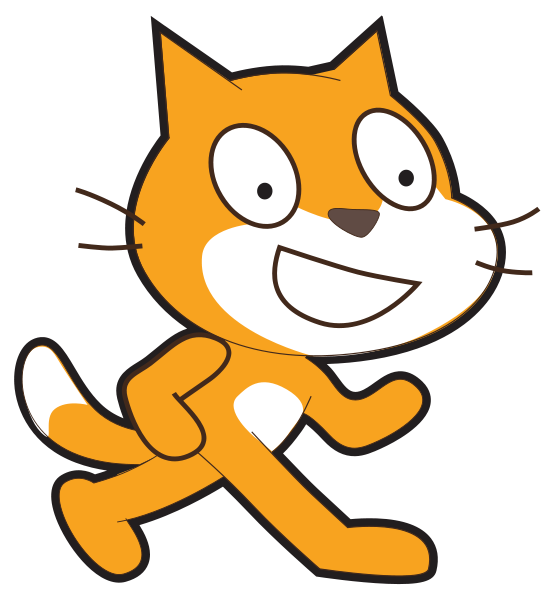# 12 digit product codes: Check if total is a multiple of 10 (one line of input)

Challenge Level: Growing experience

Learning outcomesStudents will be able to:

## Requirement:

Write a program that asks the user to enter a 12 digit product code, all in one line of input. Then adds up all the digits, every second one multiplied by 3 (1st, 3rd, and so on) and show if this total is a multiple of 10 (valid product code). For this challenge make sure the user can only enter a 12 digit product code.

## Testing examples:

Your program should display the outputs shown in this table for the given inputs provided:

Input Output
838310022524 Valid product code!
811571013579 Valid product code!
838310032524 Invalid product code!
614141000012 Valid product code!
811571023579 Invalid product code!
664141000012 Invalid product code!

## Languages

Scratch#### What it should look like

Click on the green flag, enter the inputs provided in the “testing examples” to see the expected output of your program.

Recommended blocks
when green flag clicked

ask [Enter a 12 digit product code:] and wait
set [index v] to 

set [product code v] to []

set [total 1 v] to 

set [total 2 v] to 

set [total v] to 

set [total 1 v] to ((total 1) + (letter (index) of (product code)))

change [index v] by (1)

set [total 2 v] to ((total 2) + (letter (index) of (product code)))

change [index v] by (1)

set [total v] to (((total 1) * (3)) + (total 2))

change [product code v] by (answer)
say [Valid product code!]

say [Invalid product code!]

say [Please enter a 12 digit number!]
if <(length of (product code)) = > then
else
end

repeat (6)
end

if <((total) mod (10)) = > then
else
end
Hints
• You can find the number digits in a number by using the length of () block unders “Operators”. For example: length of (293833992383) //12

• You can access a letter (or a digit) at a specified position in a string (or number) by using the letter () of [] block under “Operators”. For example: letter (4) of  //9

• In this challenge you need to access all the digits of the product code number entered, adding the 1st, 3rd, 5th and so on digits and storing the result in variable "total 1" and adding the 2nd, 4th, 6th and so on digits and storing the result in variable "total 2". The output displays if the sum of "total 1" multiplied by 3 and "total 2" is a multiple of 10 or not.